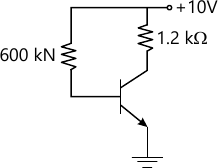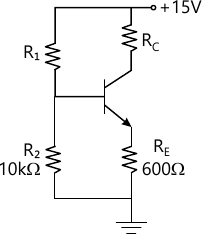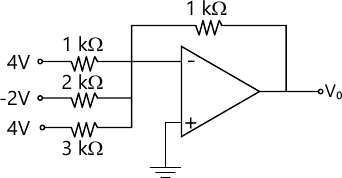MORE IN Basic Electronics
VTU First Year Engineering (C Cycle) (Semester 2)
Basic Electronics
December 2011
Total marks: --
Total time: --
INSTRUCTIONS
(1) Assume appropriate data and state your reasons
(2) Marks are given to the right of every question
(3) Draw neat diagrams wherever necessary

Choose the correct for the following :-
1 (a) (i) The voltage a which forward current through the diode starts increasing rapidly is called as _____
(A) Saturation voltage
(B) Breakover voltage
(C) cut in voltage
(D) cut off voltage
1 M
1 (a) (ii) Dynamic zener resistance is _____ in reverse breakdown condition.
(A) very high
(B) high
(C) zero
(D) very small
1 M
1 (a) (iii) Smaller the ripple factor, the output will have higher _____ components.
(A) AC
(B) DC
(C) Both AC and DC
(D) Pulse
1 M
1 (a) (iv) The transformer utilization factor of a bridge type full wave rectifier is _____
(A) 0.287
(B) 0.812
(C) 0.864
(D) 0.48
1 M
1 (b) Draw the AC equivalent circuit of a diode.
4 M
1 (c) With a circuit diagram, explain the working of a centre-tapped FWR.
6 M
1 (d) Prove that ripple factor of HWR is 1.21
6 M

Choose the correct answer for the following :-
2 (a) (i) The current conduction in bipolar junction transistor is because of _____
(A) Electrons
(B) Holes
(C) Both electrons and holes
(D) current
1 M
2 (a) (ii) In cut off region both base-to-collector and base to emitter junctions are _____
(A) forward biased
(B) ON
(C) Reverse biased
(D) None of these
1 M
2 (a) (iii) In a transistor IB=30 mA and IE=10 mA. What is the value of ? ?
(A) 0.92
(B) 0.99
(C) 0.98
(D) 0.96
1 M
2 (a) (iv) In CB-mode of a transistor when the reverse bias voltage increases, the width of depletion region also increases, which reduces the electrical base width calles as _____
(A) Depletion
(B) Early effect
(C) cut in
(D) punch through effect
1 M
2 (b) What are the advantages of trasistor over vaccum tube?
4 M
2 (c) Draw and explain the input and output characteristics of CE configuration of a transistor.
6 M
2 (d) For the CE-circuit shown in fig 2(d), draw the DC load line and obtain Q-point values Assume ?=100 and VBE=0.7 V6 M

Choose the correct answer for the following :-
3 (a) (i) Ideally stability factor should be zero to get _____ Q-point.
(A) Unstable
(B) Centre of the cutoff
(C) Stable
(D) None
1 M
3 (a) (ii) Which of the following factor affects the Q-point stability?
(A) ICO
(B) Coupling capacitor
(C) Emitter resistor
(D) Bypass capacitor
1 M
3 (a) (iii) In what biasing circuit voltage shunt negative feed back is provided?
(A) Voltage divider biasing
(B) Fixed bias
(C) Collector to base bias
(D) Emitter bias
1 M
3 (a) (iv) Fixed bias circuit provides _____ stability
(A) Poor
(B) High
(C) Better
(D) Very good
1 M
3 (b) For the circuit shown in fig. IC=2 mA, ?=100, and VCE=3V, Calculate R1 and EC. Assume VBE=0.6V.8 M
3 (c) What factors cause instability of a Q-point? Explain it.
8 M

Choose the correct answer for the following :-
4 (a) (i) JFET is a _____ device
(A) Bipolar
(B) Unipolar
(C) Uni-Bipolar
(D) None of these
1 M
4 (a) (ii) PNPN device is an _____
(A) UJT
(B) SCR
(C) MOSFET
(D) BJT
1 M
4 (a) (iii) The UJT relaxation oscillator is used to generate _____
(A) Square wave signal
(B) Rectangular wave signal
(C) Sine wave signal
(D) Triggering pulse
1 M
4 (a) (iv) The holding current in SCR is _____ latching current
(A) More than
(B) Less than
(C) Equal to
(D) None of these
1 M
4 (b) Draw the equivalent circuit of a UJT and mention its applications.
4 M
4 (c) What are the applications of SCR?
4 M
4 (d) Draw the drain characteristics of a n-channel JFET and explain it.
8 M

Choose the correct answer for the following :-
5 (a) (i) If the voltage gain of the amplifier is 0.001, what is the value of gain is dB's?
(A) -60
(B) -62
(C) 60
(D) 100
1 M
5 (a) (ii) With negative feedback, the bandwidth of an amplifier _____
Decreases
(B) Increases
(C) Both A and B
(D) Constant
1 M
5 (a) (iii) In oscillator circuit _____ feedback is used,
(A) Voltage seriers
(B) Positive
(C) Negative
(D) Both +ve and -ve
1 M
5 (a) (iv) In RC-phase shift oscillator each section of RC-network produces phase shift of _____
(A) 60°
(B) 30°
(C) 180°
(D) 90°
1 M
5 (b) With a neat diagram, explain the operation of Colpitt's oscillator.
8 M
5 (c) Explain the operation of single stage RC coupled amplifier and draw its frequency response.
8 M

Choose the correct answer for the following :-
6 (a) (i) For a differential amplifier A4=10000 and CMRR=108. What is the value of AC?
(A) =10-4
(B) 10-6
(C) 104
(D) 100
1 M
6 (a) (ii) For an inverting op-amp if R1=RF then circuit is called _____
(A) Sign changer
(B) Sign multiplier
(C) +ve sign
(D) None of these
1 M
6 (a) (iii) The ideal bandwidth of an op-amp is _____
(A) Zero
(B) Infinity
(C) High
(D) Medium
1 M
6 (a) (iv) Buffer and level shifter is usually a
(A) Current follower
(B) Collector follower
(C) Resistance follower
(D) Emitter follower
1 M
6 (b) Define the following terms with respect to op-amps
(A) Slew rate
(B) Power supply rejection ratio
(C) CMRR.
6 M
6 (c) Derive the expression of output voltage of a op-amp differentiator.
5 M
6 (d) Determine the output voltage for the op-amp adder circuit shown in fig.5 M

Choose the correct answer for the following :-
7 (a) (i) The carrier frequency is _____ modulating frequency
(A) Lower than
(B) Higher than
(C) Equal to
(D) None of these
1 M
7 (a) (ii) The bandwidth of AM waves is _____
(A) 2fm
(B) fm
(C) fm/2
(D) None of these
1 M
7 (a) (iii) Find the decimal equivalent of (10AB)16.
(A) 3267
(B) 4265
(C) 4268
(D) 4267
1 M
7 (a) (iv) What is the binary equivalent of (1126)8?
(A) 001 001 010 110
(B) 100 001 010 110
(C) 110 110 001 001
(D) 001 001 110 010
1 M
7 (b) Draw the block diagram of superheterodyne receiver and explain the function of each block.
8 M
7 (c) Convert (BCDE)16= ( )2=( )8=( )10.
3 M
7 (d) Subtract (57)10 from (43)10 2's complement form.
5 M

Choose the correct answer for the following :-
8 (a) (i) For NAND-Gate both inputs are high, then output will be _____
(A) High
(B) Low
(C) Tristate
(D) None of these
1 M
8 (a) (ii) Y=AB+AB is a Boolean expression for
(A) EX-OR
(B) EX-NAND
(C) EX-NOR
(D) None of these
1 M
8 (a) (iii) A+(B+C)=(A+B)+C is a _____ property
(A) Associative
(B) Commutative
(C) Distributive
(D) None of these
1 M
8 (a) (iv) The expression Y=AB+BC+BC when simplified is _____
(A) B+C
(B) AB
(C) A+B
(D) AB+C
1 M
8 (b) Simplify the following Boolean expression
Y=ABC+ABC+ABC+ABC
Y=(A B+AC)(BC+B C)(ABC)
6 M
8 (c) Draw the logic circuit of a full adder and also write its truth table with sum and carry expressions.
6 M
8 (d) Realize the expression F=(X+Y(Z+Y)) using only NAND-Gates.
4 M

More question papers from Basic Electronics